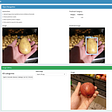# Cats and Dogs Classifier — Easiest Way— with source code— Easy Explanation

In today’s blog, we will be building a Cats and Dogs Classifier using Convolutional Neural Networks. This is going to be a very fun blog, so without any further due.

# Let’s do it…

## Step 1 — Importing required libraries for Cats and Dogs Classifier.

`import numpy as npimport pandas as pd from keras.preprocessing.image import ImageDataGenerator, load_imgfrom keras.utils.np_utils import to_categoricalfrom sklearn.model_selection import train_test_splitimport matplotlib.pyplot as pltimport randomimport osfrom keras.models import Sequentialfrom keras.layers import Conv2D, MaxPooling2D, Dropout, Flatten, Dense, Activation, BatchNormalizationfrom keras.callbacks import EarlyStopping, ReduceLROnPlateauimport cv2`

## Step 2 — Initializing some constants.

`IMAGE_WIDTH=128IMAGE_HEIGHT=128IMAGE_SIZE=(IMAGE_WIDTH, IMAGE_HEIGHT)IMAGE_CHANNELS=3`

## Step 3 — Loading input data for Cats and Dogs Classifier.

`filenames = os.listdir("train")categories = []for filename in filenames:    category = filename.split('.')    if category == 'dog':        categories.append(1)    else:        categories.append(0)df = pd.DataFrame({    'filename': filenames,    'category': categories})`
• We have all our images in the train folder. So os.listdir(‘train’) will give a list of all image names.
• Then we are just traversing in all images and extracting their category (dog or cat) from image names.
• Finally, we are creating a dataframe of our data.

## Step 4 — Checking the head of the data.

`df.head()`

## Step 5 — Checking the tail of the data.

`df.tail()`

## Step 6 — Visualizing the category column.

`df[‘category’].value_counts().plot.bar()`

## Step 7 — Building the model for Cats and Dogs Classifier.

`model = Sequential()model.add(Conv2D(32, (3, 3), activation='relu', input_shape=(IMAGE_WIDTH, IMAGE_HEIGHT, IMAGE_CHANNELS)))model.add(BatchNormalization())model.add(MaxPooling2D(pool_size=(2, 2)))model.add(Dropout(0.25))model.add(Conv2D(64, (3, 3), activation='relu'))model.add(BatchNormalization())model.add(MaxPooling2D(pool_size=(2, 2)))model.add(Dropout(0.25))model.add(Conv2D(128, (3, 3), activation='relu'))model.add(BatchNormalization())model.add(MaxPooling2D(pool_size=(2, 2)))model.add(Dropout(0.25))model.add(Flatten())model.add(Dense(512, activation='relu'))model.add(BatchNormalization())model.add(Dropout(0.5))model.add(Dense(2, activation='softmax')) # 2 because we have cat and dog classesmodel.compile(loss='categorical_crossentropy', optimizer='rmsprop', metrics=['accuracy'])model.summary()`
• Here we have created a sequential Keras model which is very easy to use. Just use model.add() and keep on adding layers as per your use case’s convenience.
• Here we have basically used 3 sets of Conv2D BatchNormalization Maxpooling Dropout layers.
• And after that, we flattened the results from these layers and passed on those results to Dense layers or Fully connected layers.
• The last Dense layer would always contain n no. of nodes where n is the no. of classes in the dataset.
• The last step in building the model is model.compile() which is used to put together everything we did above.
• We use categorical cross-entropy here because we have 2 categories here, we used rmsprop optimizer, we could have also used adam but rmsprop was giving better results in this case, and the metrics on what we will measure the performance of our model is accuracy.

## Step 8 — Initializing Callbacks for Cats and Dogs Classifier model.

`earlystop = EarlyStopping(patience=10)learning_rate_reduction = ReduceLROnPlateau(monitor='val_accuracy',                                             patience=2,                                             verbose=1,                                             factor=0.5,                                             min_lr=0.00001)callbacks = [earlystop, learning_rate_reduction]`
• Simply initializing EarlyStopping and ReduceLROnPlateau here.
• EarlyStopping stops the training earlier when val_accuracy stops increasing or val_loss stops decreasing.
• ReduceLROnPlateau reduces the learning rate when our model reaches near minima of the loss function.

## Step 9 — Replacing 0s with cat and 1s with dog.

`df[“category”] = df[“category”].replace({0: ‘cat’, 1: ‘dog’})`

## Step 10 — Split the data into train and validation.

`train_df, validate_df = train_test_split(df, test_size=0.20, random_state=42)train_df = train_df.reset_index(drop=True)validate_df = validate_df.reset_index(drop=True)`

## Step 11 — Counts of both categories in train data.

`train_df[‘category’].value_counts().plot.bar()`
• Both 0 and 1 have 10000 images each in the training dataset.

## Step 12 — Counts of both categories in validation data.

`validate_df[‘category’].value_counts().plot.bar()`
• Both 0 and 1 have 2500 images each in validation dataset.

## Step 13 — Getting some shapes.

`total_train = train_df.shapetotal_validate = validate_df.shapebatch_size=15`
• First-line gives us the no. of images in the training dataset.
• Second-line gives us the no. of images in the validation dataset.

## Step 14 — Augmenting training data of Cats and Dogs Classifier.

`train_datagen = ImageDataGenerator(    rotation_range=15,    rescale=1./255,    shear_range=0.1,    zoom_range=0.2,    horizontal_flip=True,    width_shift_range=0.1,    height_shift_range=0.1)train_generator = train_datagen.flow_from_dataframe(    train_df,     "train/",     x_col='filename',    y_col='category',    target_size=IMAGE_SIZE,    class_mode='categorical',    batch_size=batch_size)`

## Step 15 — Augmenting validation data.

`validation_datagen = ImageDataGenerator(rescale=1./255)validation_generator = validation_datagen.flow_from_dataframe(    validate_df,     "train/",     x_col='filename',    y_col='category',    target_size=IMAGE_SIZE,    class_mode='categorical',    batch_size=batch_size)`

## Step 16 — Visualizing Augmentation on a random example image.

`example_df = train_df.sample(n=1).reset_index(drop=True)example_generator = train_datagen.flow_from_dataframe(    example_df,     "train/",     x_col='filename',    y_col='category',    target_size=IMAGE_SIZE,    class_mode='categorical')plt.figure(figsize=(12, 12))for i in range(0, 15):    plt.subplot(5, 3, i+1)    for X_batch, Y_batch in example_generator:        image = X_batch        plt.imshow(image)        breakplt.tight_layout()plt.show()`

## Step 17 — Training and saving our Cats and Dogs Classifier model.

`epochs = 50history = model.fit_generator(    train_generator,     epochs=epochs,    validation_data=validation_generator,    validation_steps=total_validate//batch_size,    steps_per_epoch=total_train//batch_size,    callbacks=callbacks)model.save("model.h5")`

## Step 18 — Visualizing the training process.

`fig, (ax1, ax2) = plt.subplots(1, 2, figsize=(12, 6))ax1.plot(history.history['loss'], color='b', label="Training loss")ax1.plot(history.history['val_loss'], color='r', label="validation loss")ax1.set_xticks(np.arange(1, epochs, 1))ax1.set_yticks(np.arange(0, 1, 0.1))ax2.plot(history.history['accuracy'], color='b', label="Training accuracy")ax2.plot(history.history['val_accuracy'], color='r',label="Validation accuracy")ax2.set_xticks(np.arange(1, epochs, 1))legend = plt.legend(loc='best', shadow=True)plt.tight_layout()plt.show()`

## Step 19 — Live predictions of Cats and Dogs Classifier.

`for i in range(10):    all_test_images = os.listdir('test')    random_image = random.choice(all_test_images)    img = cv2.imread(f'test/{random_image}')    img = cv2.resize(img,(IMAGE_HEIGHT,IMAGE_WIDTH))        org = img.copy()    img = img.reshape(1,128,128,3)        pred = model.predict(img)    print(['cat','dog'][int(pred)])    cv2.imshow('Live predictions',org)    cv2.waitKey(0)cv2.destroyAllWindows()`
• Randomly take out 10 images from the test folder and make predictions on them.

Do let me know if there’s any query regarding Cats and Dogs Classifier by contacting me on email or LinkedIn.

To explore more Machine Learning, Deep Learning, Computer Vision, NLP, Flask Projects visit my blog — Machine Learning Projects

For further code explanation and source code visit here https://machinelearningprojects.net/cats-and-dogs-classifier/

So this is all for this blog folks, thanks for reading it and I hope you are taking something with you after reading this and till the next time 👋…

Read my previous post: DIMENSIONALITY REDUCTION USING AUTOENCODERS

--

--

--

## More from MLearning.ai

Data Scientists must think like an artist when finding a solution when creating a piece of code. ⚪️ Artists enjoy working on interesting problems, even if there is no obvious answer ⚪️ linktr.ee/mlearning 🔵 Follow to join our 18K+ Unique DAILY Readers 🟠

## ML in Finance, specifically Backtesting## Review: U-Net: Convolutional Networks for (Biomedical Image Segmentation)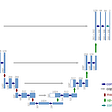## Detect single neuronal cells in microscopy images 🦠🔬👩🏻‍⚕️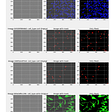## Fundamental OMachine Learning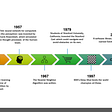## BLURRINGS IN CV2 — SIMPLE BLUR, BOX BLUR, GAUSSIAN BLUR, AND MEDIAN BLUR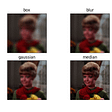## Modelizing a tennis match with Markov Chains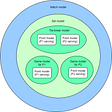## Building an Image Search System with AnalyticDB## Abhishek Sharma

Data Scientist || Blogger || machinelearningprojects.net

## Deep Convolutional Neural Networks (DCNNs) explained in layman's terms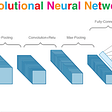## HARRY’S INVISIBILITY CLOAK — LESS THAN 50 LINES OF CODE — WITH SOURCE CODE — EASIEST EXPLANATION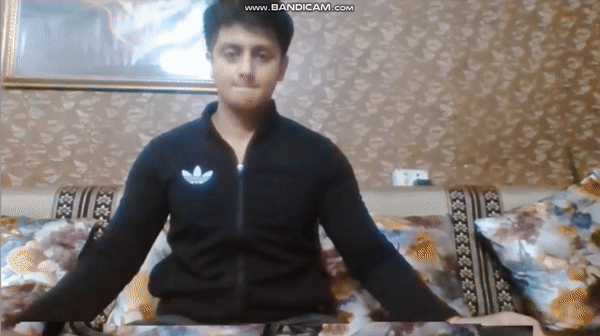## Building A Deep Learning-Based Object Detection App Using R Shiny and Tensorflow